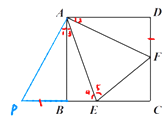# 【提招专题】全等三角形 模型6——半角模型

【条件】如图两个角共顶点，其中一个角（45º）是另一个角（90º）的一半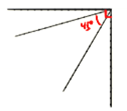【结论】EF=BE+DF  EA平分BEFFA平分DFE

③△EFC的周长等于正方形边长的2④ 如图：AM=AB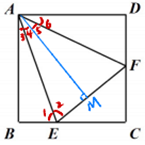⑤  如图：EAF=45º，则EF²=BE²+FC²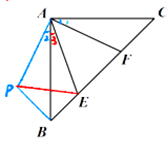【证明】延长CB至点P，使得BP=DF 连接AP

第一次全等                                      第二次全等

（3）BP=DF（构造）                       （3）AE=AE

AP=AF 1=2                           PE=EF

∵∠2+3=45º                                    PB+BE=EF

∴∠1+3=45º,                                   DF+BE =EF

∴∠PAE=FAE

②  得：AEP≌△AEF，则4=5AFE=P

又APB≌△AFD∴∠P=AFD∴∠AFE=AFD

∴EA平分BEFFA平分DFE

③  得：EF=BE+DF∴△EFC的周长＝EF+EC+CFBE+DF+EC+CF

=BC+DC ∴△EFC的周长等于正方形边长的2

AAMEF，则AME=B=90º。由1=2AE=AE

∴△ABE≌△AMEAAS），AM=AB

⑤ 如图，过点AAPAF AP=AF.连接PE

∵∠CAB= ∠PAF=90º，∠1=∠2

第一次全等                      第二次全等

在ABPACF              AEPAEF

（1）AB=AC                        （1）  AP=AF

（2） 2=1                       （2）PAE=FAE

（3）AP=AF                        （3） AE=AE

ABP≌△ACFSAS         ∴△AEP≌△AEFSAS

∴BP=CF ABP=C=45º    PE=EF

∵∠EAF=45º                               RtPBE中，PE²=PB²+BE²

∴∠1+3=45º,                           EF²=CF²+BE²

∴∠2+3 =45º

【条件】  如图，△ABC是等边三角形，△BDC 是等腰三角形，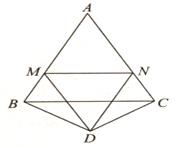【结论】MN= BM+CN;

②△MAN 的周长等于ABC边长的 2 ;

③MDBMN的平分线，NDCNM的平分线

【证明】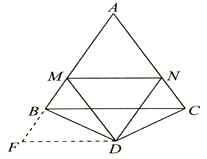∵△BDC是等腰三角形，且∠BDC=120°，

∴∠BCD=∠DBC=30°.

∵△ABC是等边三角形，∴∠ABC = ∠BAC = ∠BCA=60°,

∴∠DBA= ∠DCA=90°.

∴∠BDF=∠CDN,∠F=∠CND,DF=DN.

∵∠MDN=60°, ∴∠BDM+∠CDN=60°,∴∠BDM+∠BDF=60°，

∴△DMN≌△DMFSAS），∴ MN=MF=BM+CN,

F=∠MND=∠CND，∠FMD=∠DMN

∴△AMN的周长是 AMANMN=AMMBCNAN=ABAC=2边长.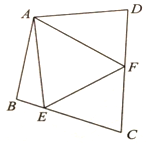【结论】EF=BE+FD;